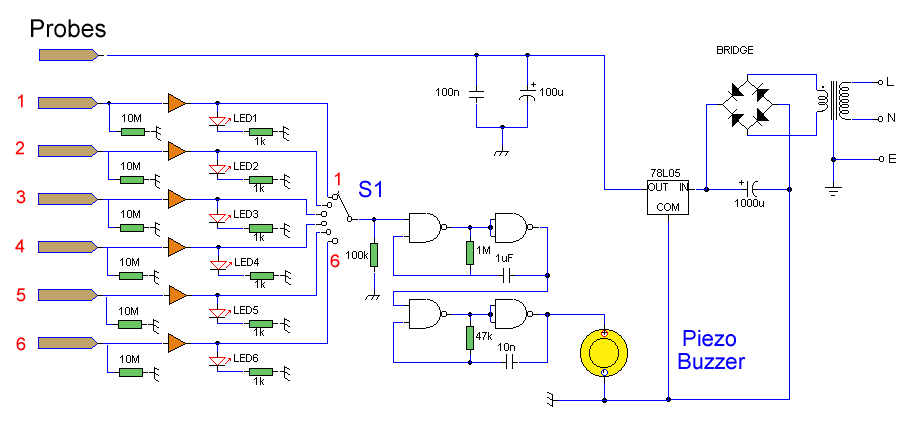# Water Level Alarm

Andy Collinson

Description
A circuit that offers visual indication of fluid level in a vessel, with a switchable audible alarm. Example uses would be to monitor the level of water in a bath or cold storage tank.Click, for enlarge

The Conductance of Fluids
Conductance is the reciprocal of resistance. The conductance of fluids vary with temperature, volume and separation distance of the measurement probes. Tap water has a conductance of about 50 uS / cm measured at 25°C. This is 20k/cm at 25°C. See this site for more details about the conductance of fluids.

Circuit Notes
This circuit will trigger with any fluid with a resistance under 900K between the maximum separation distance of the probes. Let me explain further. The circuit uses a 4050B CMOS hex buffer working on a 5 volt supply. All gates are biased off by the 10M resistors connected between ground and buffer input. The "common" probe the topmost probe above probe 1 in the diagram above is connected to the positive 5 volt supply. If probe 1 is spaced 1cm away from the common probe and tap water at 25°C is detected between the probes (a resistance of 20k) then the top gate is activated and the LED 1 will light. Similarly if probe 2 at 2 cm distance from the common probe detects water, LED 2 will light and so on. Switch 1 is used to select which output from the hex buffer will trigger the audible oscillator made from the gates of a CMOS 4011B IC.

Placement of Probes
As 7 wires are needed for the probe I recommend the use of 8 way computer ribbon cable. The first two wires may be doubled and act as the common probe wire. Each subsequent wire may be cut to required length, if required a couple of millimeters of insulation may be stripped back, though the open "cut off" wire end should be sufficient to act as the probe. The fluid and distance between probe 6 and the common probe wire must be less than 900k. This is because any voltage below 0.5 Volt is detected by the CMOS IC as logic 0. A quick potential check using a 900k resistance and the divider formed with the 10M resistor at the input proves this point:

5 x (0.9 / (0.9+10) = 0.41 V

As this voltage is below 0.5 volt it is interpreted as a logic 0 and the LED will light. If measuring tap water at 25°C then the distance between top probe and common may be up to 45 cm apart. For other temperatures and fluids, it is advisable to use an ohmmeter first. When placing the probes the common probe must be the lowest placed probe, as the water level rises, it will first pass probe 1, then 2 and finally probe 6.

zen22142.zen.co.uk